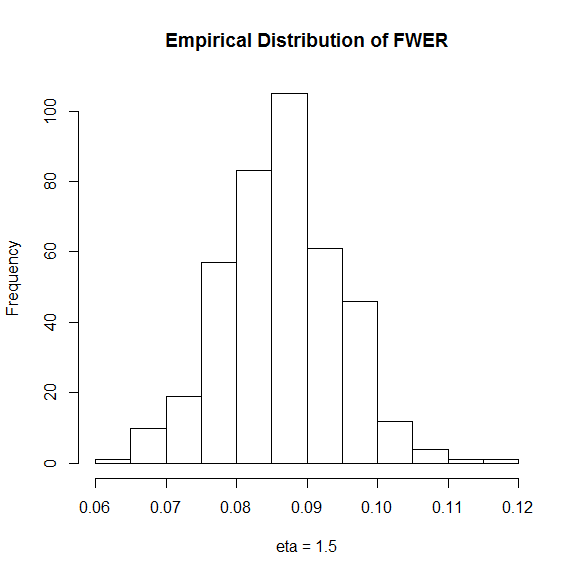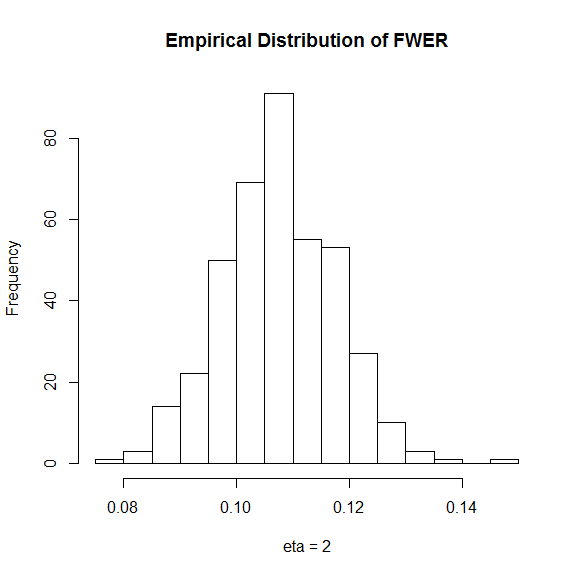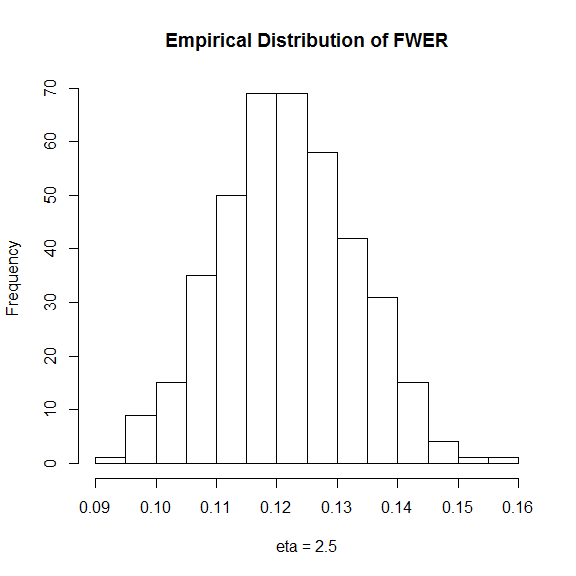# Using the FWER controlling support test procedure of Stange, Bodnar, Dickhaus (2015)

#### 2019-01-21

This vignette demonstrates the usage of the FWER controlling support test described in J. Stange, T. Bodnar and T. Dickhaus (2015). The paper is available at https://doi.org/10.1007/s10182-014-0241-5. All following references to equations and theorems refer to this paper.

## Performing FWER controlling support tests using fwer.support_test

Let $$X_1,\cdots,X_n$$ denote an i.i.d. sample which has the stochastic representation $$X_{i,j}=\vartheta_j Z_j$$ where $$Z_j$$ is a random variable which takes values in $$[0,1]$$ and which is distributed according to a Gumble copula with Beta margins. Then the test simultaneously tests the hypotheses $$H_{0,j}: \vartheta_j \le \vartheta_j^*$$ versus the corresponding alternatives $$H_{1,j}: \vartheta_j>\vartheta_j^*$$.

For values drawn from this model the test can be performed using fwer.support_test as follows:

set.seed(0)
sample_GumbelBeta<-function(m,n,eta,alpha,beta,theta)
{
V<-stabledist::rstable(n,1/eta,1,(cos(pi/(2*eta)))^eta,as.numeric(eta==1),1) #vector of stable distributed numbers
UU<-matrix(rexp(n*m),nrow=n,ncol=m)                         #returns matrix of exponentially distributed numbers m columns, n rows
sample_gumbel<-exp(-(UU/V)^(1/eta))                         #each row is divided by the same number v, then "psi" is applied
sample_gumbel_beta0<-stats::qbeta(sample_gumbel,alpha,beta) #transform to beta margins
return( sample_gumbel_beta0%*%diag(theta)  )                #return columns multiplied by respective scale theta
}
n <- 100
alpha <- 3
beta <- 3
m <- 10
m0 <- 5
theta <- c(rep(2,m0),2+runif(m-m0,max=0.5))
sample <- sample_GumbelBeta(m,n,1,alpha,beta,theta)
res <- fwer.support_test(sample,rep(2,m),alpha,beta)
res$test$Empirical
#>   FALSE FALSE FALSE FALSE FALSE  TRUE  TRUE FALSE  TRUE  TRUE

Thus only the hypothesis $$H_{0,8}$$ is wrongly not rejected.

## Figures 6 and 7: Empirical power and FWER for model 2a) (Gumbel copula with Beta margins)

The power and FWER of the procedure under model 2a) are estimated using a Monte carlo simulation. To this end samples are repeatedly drawn from a Gumbel copula with Beta margins

samplefun <- function(theta) sample_GumbelBeta(m,n,eta,alpha,beta,theta)

and then the test is applied:

testfun <- function(X) fwer.support_test(X,rep(2,m),alpha,beta)

To see the full code for generating the figures execute the following code:

v <- vignette('fwer-support-test',package = 'MHTcop')
file.edit(paste(v$Dir,'doc',v$R,sep='/'))

### Figure 6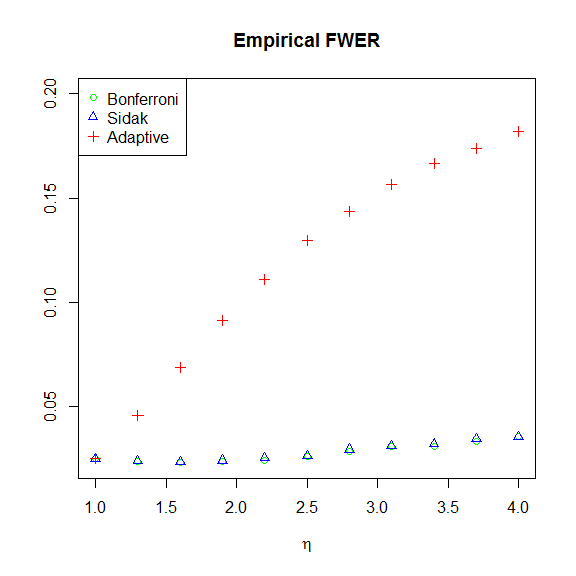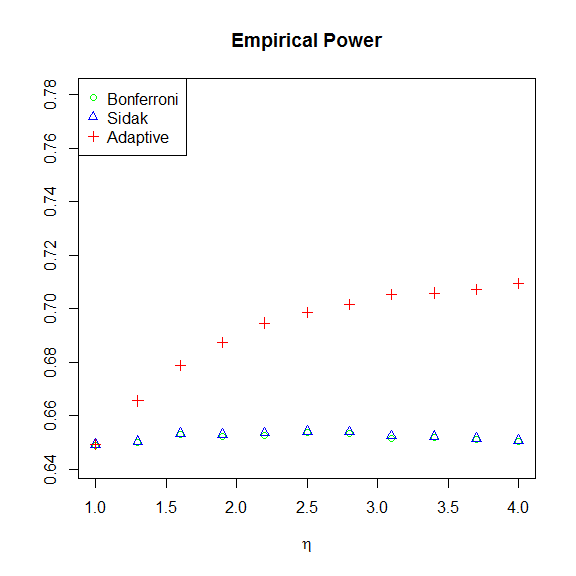### Figure 7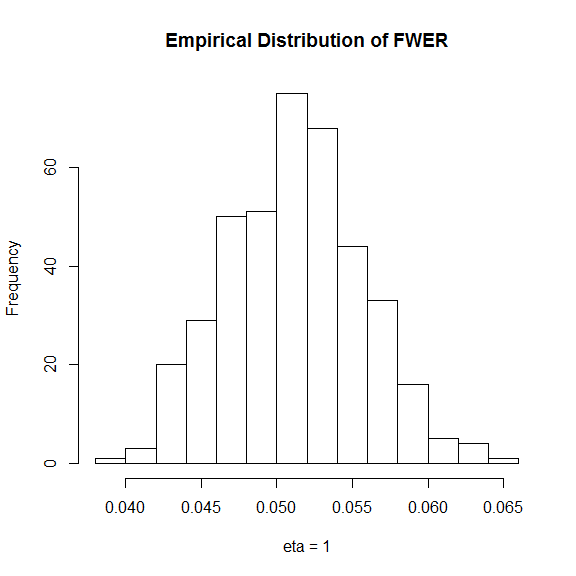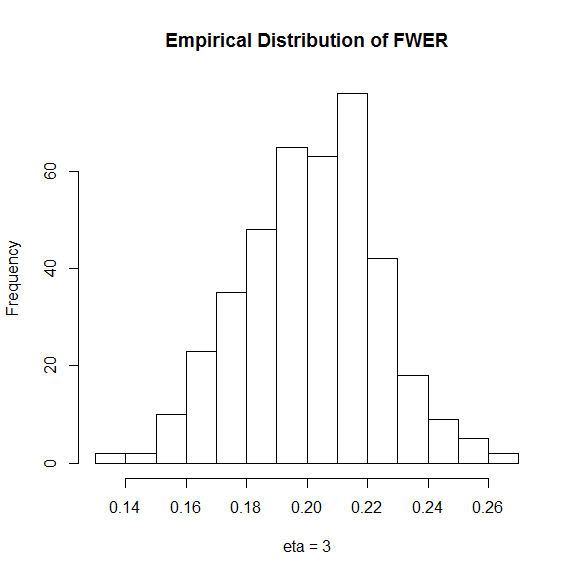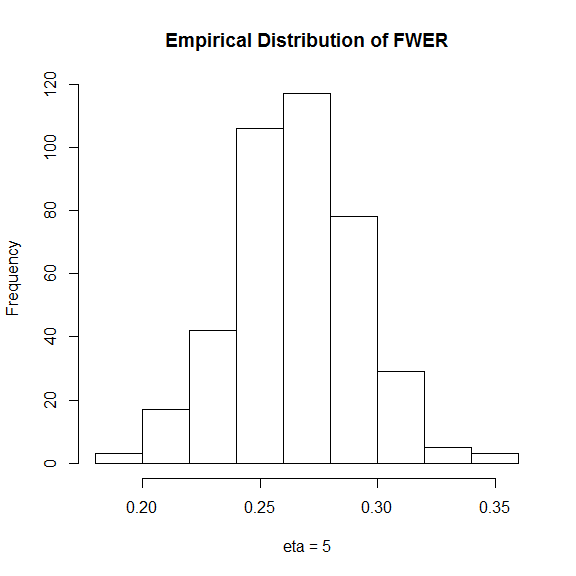## Figures 8 and 9: Empirical power and FWER for model 2b) (Archmidean copula defined by equation (18))

The power and FWER of the procedure under model 2b) are estimated using a Monte carlo simulation. To this end samples are repeatedly drawn from the copula defined by (18)

# Sampling from the Archimedean copula defined by (18)
sample_arch_copula <- function(m,n,theta,eta,alpha,beta)
{
FR <- function(x,d,eta) {
g <- numeric(length=d)
x_eta <- x^eta
g <- 1/(1+x_eta)
i <- 0:(d-1)
p_ <- cumprod((eta-i)/(i+1))
for(k in 1:(d-1)) {
g[k+1] <- -1 * x_eta * sum(g[1:k] * p_[k:1]) / (1+x_eta)
}
sgn <- rep_len(c(1,-1),d)
sgn[d] <- sgn[d] * (g[d]>0)
return(1 - sum(sgn*g))
}

RadialCDF <- function(t,eta,dim=4) {
eta<-1/eta
x<-tan(pi/2*t)
return(FR(x,dim,eta))
}
psi<-function(t) 1/(t^(1/eta)+1)
T<-runif(n,max=delta)
#define help function for inversion sampling
inverter<-function(x) uniroot(function(t) toinvert(t,x),interval=c(0,1),tol=.Machine$double.eps)$root
W<-tan(pi/2*sapply(T,inverter))
#draw sample uniformly on d-simplex
S<-MCMCpack::rdirichlet(n,alpha=rep(1,m))
#random vectors that follow copula with uniform margins
copula_plain<-psi(S*W)   #n rows and m columns
#transform to beta margins
copula_withBeta<-qbeta(copula_plain,alpha,beta)
#return columns multiplied by respective scale theta
return(copula_withBeta%*%diag(theta))
}
samplefun <- function(theta) sample_arch_copula(m,n,theta,eta,alpha,beta)

and then the test is applied:

testfun <- function(X) fwer.support_test(X,rep(2,m),alpha,beta,boot.reps=400)

Note that the extra parameter boot.reps. This is necessary since the copula is only in the domain of attraction of a Gumbel copula, but not a Gumbel copula and therefore the non-bootstrapped parameter estimate would not be consistent.

### Figure 8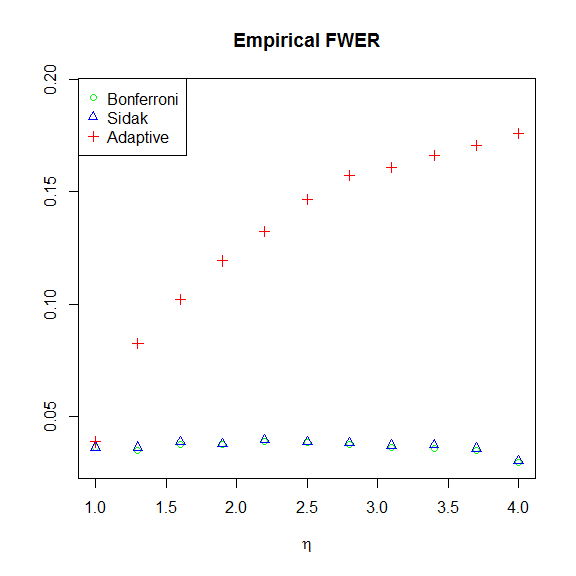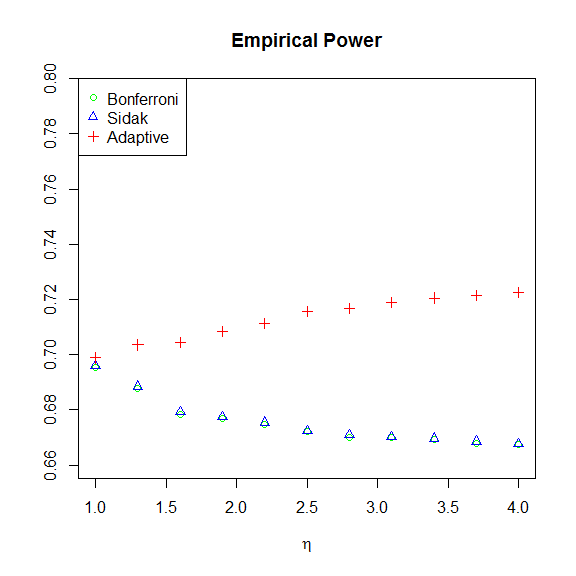### Figure 9